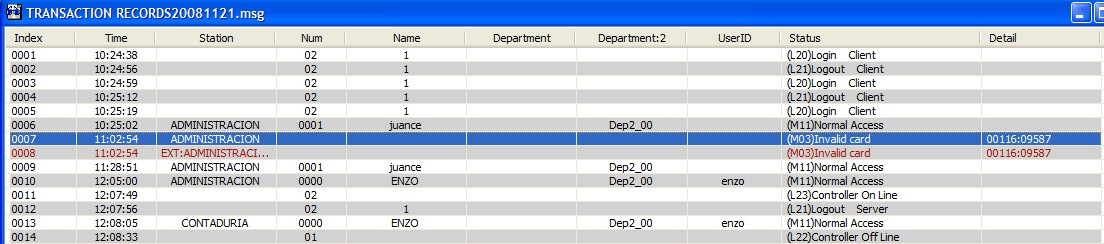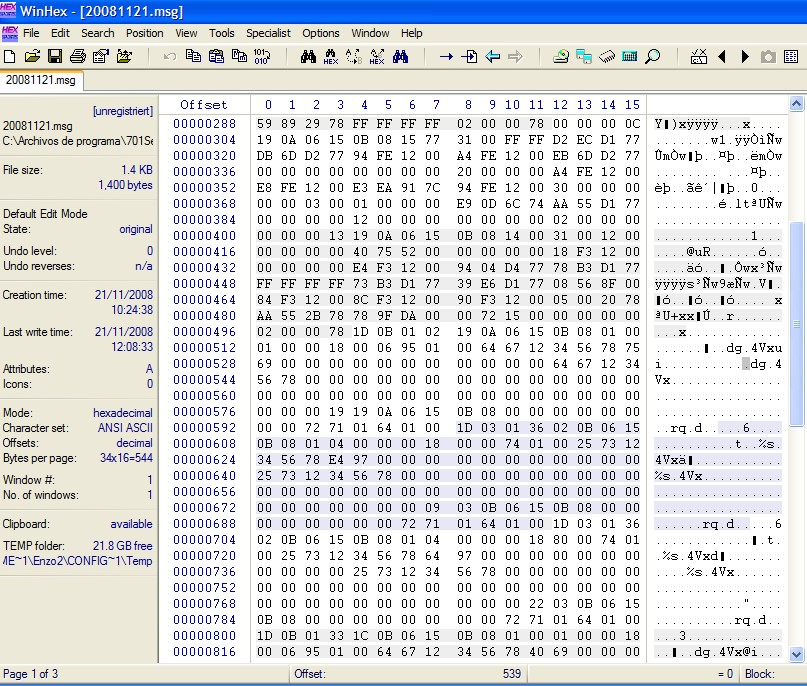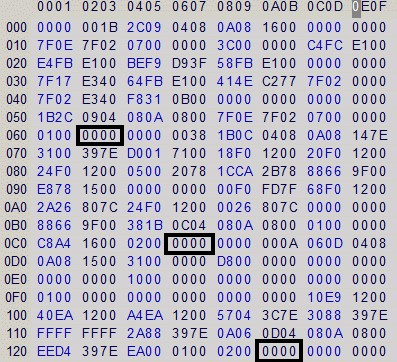# SOYAL TECHNOLOGY CO., LTD

Browsing Records
How to interpret the structure of message file?
2021-07-09
No. S00903001-EN

Serial NO : S00903001-EN

Date of Editing :  2008-12-26

Questions : How to interpret the structure of message file?

Problem Solving :

The data length is set to a fixed 100 bytes and the first two values of data structure: leading code 7E and data length will not be shown on the file.

Taking the message of the invalid card 00116:09587 as pictured and calculating where a message will start on the 2nd image as below.The formula is described as follows:

(The message you want to get -1) x 100 = value

Convert the value to HEX if data structure monitor is based on hexadecimal converter = where the message starts

i.e. the 7th message:

(7-1) x 100 = 600 (no need to convert to HEX as the monitor here is based on decimal)

The 7th message starts from 1D 03 01 ... (the message being greyed out, starts at the intersection of row 592 and column 8)

Or another way to calculate where a message stops and the formula is described as follows:

The message you want to get x 100 = value

Convert the value to HEX - 1 = where the message stops

i.e. the first message:

1x100=100

Convert to HEX -1 = 63

The first message stops at the intersection of row 060 and column 03.

i.e. the 2nd message:

2x100=200

Convert to HEX -1 = C7

The 2nd message stops at the intersection of row 0C0 and column 07

The example is to reason by analogy.Browsing Records
Go Top